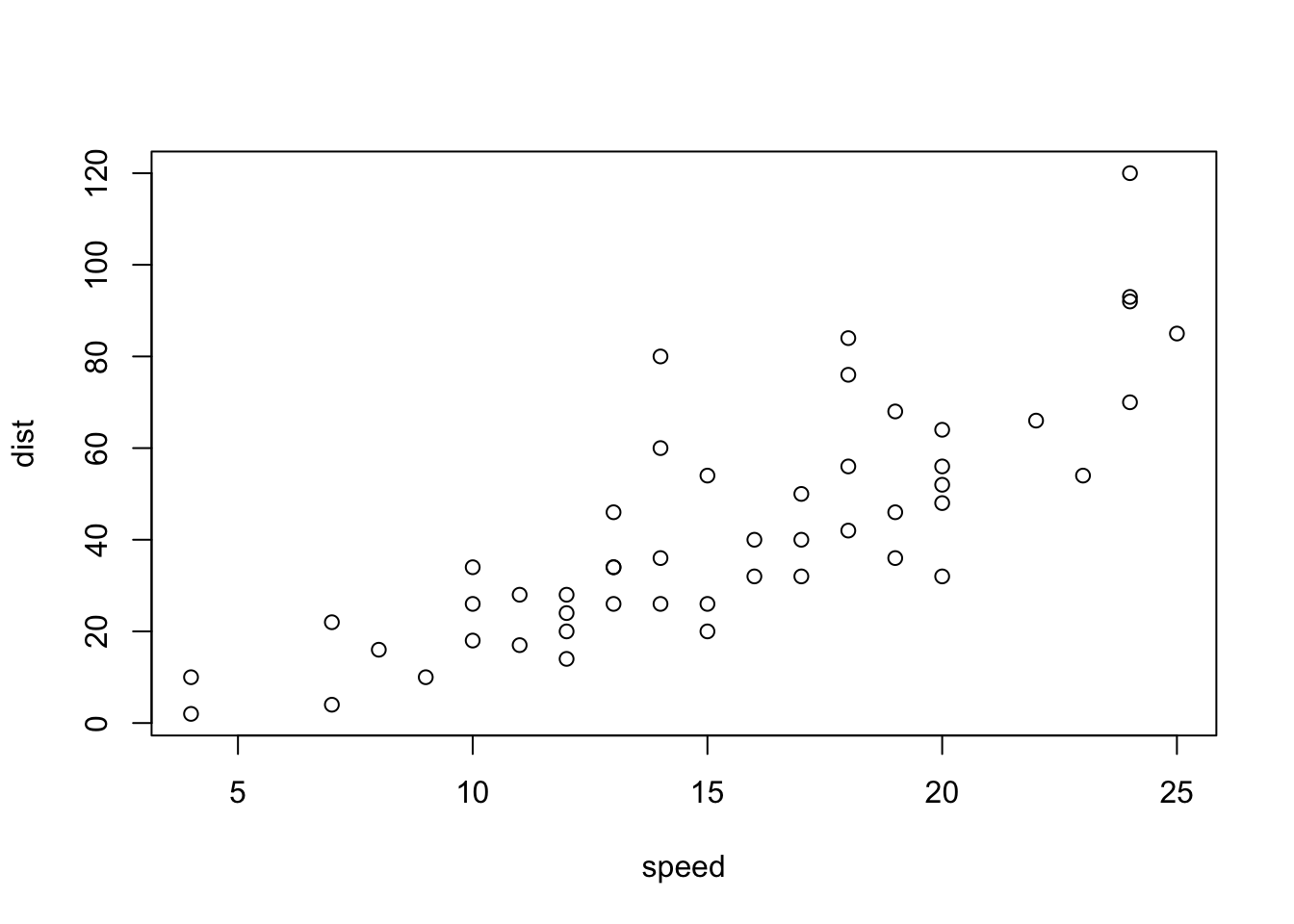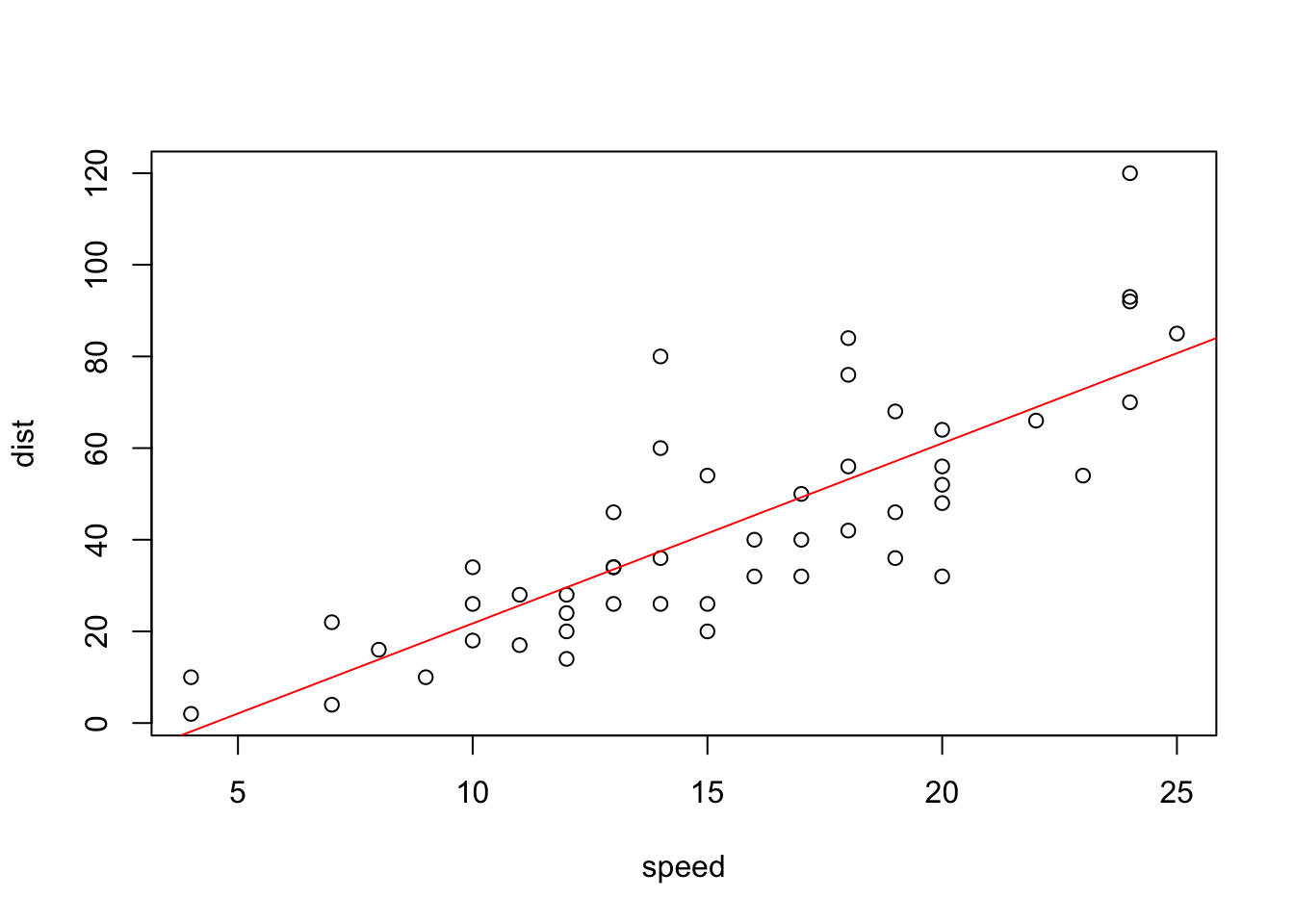In this notebook, we show how to do … well, more data stuff using R.

The selection of problems is still not intended to be complete, but it provides a gloss of the myriad ways to approach data analysis with R. Some of it overlaps with the other Data Analysis Short Course notebooks.

As always, do not hesitate to consult online resources for more examples (StackOverflow, R-bloggers, etc.).

## 1. A SIMPLE EXAMPLE

We start by loading one of the standard pedagogical datasets used with R.

data(cars)

It’s a data frame with two variables, speed and dist.

str(cars)
## 'data.frame':    50 obs. of  2 variables:
##  $speed: num 4 4 7 7 8 9 10 10 10 11 ... ##$ dist : num  2 10 4 22 16 10 18 26 34 17 ...
summary(cars)
##      speed           dist
##  Min.   : 4.0   Min.   :  2.00
##  1st Qu.:12.0   1st Qu.: 26.00
##  Median :15.0   Median : 36.00
##  Mean   :15.4   Mean   : 42.98
##  3rd Qu.:19.0   3rd Qu.: 56.00
##  Max.   :25.0   Max.   :120.00

We can compute the mean of each variable using the pre-built function colMeans().

colMeans(cars)
## speed  dist
## 15.40 42.98

But there is no such function to compute the minimum/maximum of each variable. instead, we use loops.

# min
for(i in 1:2){
print(min(cars[,i]))
}
##  4
##  2
# max
for(i in 1:2){
print(max(cars[,i]))
}
##  25
##  120

The use of loops was justified because the dataset is small, but it would be useful to know how to “vectorize” the computation using once of the *apply functions.

sapply(cars, min)
## speed  dist
##     4     2
sapply(cars, max)
## speed  dist
##    25   120
sapply(cars, mean)
## speed  dist
## 15.40 42.98

Note that in each of the three cases, the result is a data frame.

A dataset with 2 variables is easy to display.

plot(cars)We can see that the relationship between speed and distance has a strong linear component, which we can identify by running a linear regression (implemented in the function lm)

reg <- lm(dist ~ speed, data=cars)

reg is an object (the model) which is obtained when running lm(). We can summarize it and see what its attributes are as follows:

summary(reg)
##
## Call:
## lm(formula = dist ~ speed, data = cars)
##
## Residuals:
##     Min      1Q  Median      3Q     Max
## -29.069  -9.525  -2.272   9.215  43.201
##
## Coefficients:
##             Estimate Std. Error t value Pr(>|t|)
## (Intercept) -17.5791     6.7584  -2.601   0.0123 *
## speed         3.9324     0.4155   9.464 1.49e-12 ***
## ---
## Signif. codes:  0 '***' 0.001 '**' 0.01 '*' 0.05 '.' 0.1 ' ' 1
##
## Residual standard error: 15.38 on 48 degrees of freedom
## Multiple R-squared:  0.6511, Adjusted R-squared:  0.6438
## F-statistic: 89.57 on 1 and 48 DF,  p-value: 1.49e-12
attributes(reg)
## $names ##  "coefficients" "residuals" "effects" "rank" ##  "fitted.values" "assign" "qr" "df.residual" ##  "xlevels" "call" "terms" "model" ## ##$class
##  "lm"

We can extract the coefficients of the linear model as follows:

reg$coefficients ## (Intercept) speed ## -17.579095 3.932409 and use them to plot the line of best fit over the display: plot(cars) abline(reg$coefficients, col="red")## 2. WORKING WITH MATRICES

While R can work with matrices (and multi-dimensional arrays), the notation is not the most natural, as we will see presently.

Let’s generate 2 matrices that we will use in the following code blocks (remember, the brackets around the lines of code mean that the object assignment is automatically followed by an object display).

(M <- matrix(1:12, nrow=3, ncol=4))
##      [,1] [,2] [,3] [,4]
## [1,]    1    4    7   10
## [2,]    2    5    8   11
## [3,]    3    6    9   12
(V <- matrix( runif(4), 4, 1))
##            [,1]
## [1,] 0.65109487
## [2,] 0.06662291
## [3,] 0.55260257
## [4,] 0.61843535

Note that when ncol and nrow are not specified, the default parameter ordering takes over.

Since $$M$$ is $$3 \times 4$$ and $$V$$ is $$4 \times 1$$, the product $$MV$$ exists (and is $$3\times 1$$).

# use %*% to multiply matrices
M %*% V
##          [,1]
## [1,] 10.97016
## [2,] 12.85891
## [3,] 14.74767

The product $$VM$$ does not exist, however, as the dimensions are not compatible.

# uncomment the following line to see the error message
# V %*% M

The choice of %*% for matrix multiplication is perhaps less than inspired, as multiple languages use *.

What DOES * do in R?

M * 2
##      [,1] [,2] [,3] [,4]
## [1,]    2    8   14   20
## [2,]    4   10   16   22
## [3,]    6   12   18   24

Hah: multiplication by a scalar. Good to know.

One of R’s most nefarious habit is that it is not always compatible with sound mathematical notation. What do you think the following line of code does?

M * c(2, -2)
##      [,1] [,2] [,3] [,4]
## [1,]    2   -8   14  -20
## [2,]   -4   10  -16   22
## [3,]    6  -12   18  -24

Apparently, it cycles through the arguments?!? It’s hard to imagine why this construction should yield a result without breaking down, and yet it does. Beware, then. Probably not a bad idea to verify that code does what it’s supposed to do along the way.

Other familiar operations like the transpose are easy to compute:

t(M)
##      [,1] [,2] [,3]
## [1,]    1    2    3
## [2,]    4    5    6
## [3,]    7    8    9
## [4,]   10   11   12

is indeed the $$4\times 3$$ transpose of $$M$$.

Matrix manipulation is also possible. For instance, rbind and cbind are used to bind rows and columns, respectively.

cbind(t(M), V)
##      [,1] [,2] [,3]       [,4]
## [1,]    1    2    3 0.65109487
## [2,]    4    5    6 0.06662291
## [3,]    7    8    9 0.55260257
## [4,]   10   11   12 0.61843535
rbind(M,t(V))
##           [,1]       [,2]      [,3]       [,4]
## [1,] 1.0000000 4.00000000 7.0000000 10.0000000
## [2,] 2.0000000 5.00000000 8.0000000 11.0000000
## [3,] 3.0000000 6.00000000 9.0000000 12.0000000
## [4,] 0.6510949 0.06662291 0.5526026  0.6184354
# but this one wont work because of dimension incompatibility; uncomment to test
# cbind(M, V)

Practice will make it easier to remember what each operator does.

## 3. WORKING WITH STRINGS

What is usually called a string in other programming languages is a character object in R.

Character vectors are created by using double quotes (", preferred) or single quotes (', acceptable as well).

"Come on, everybody!"
##  "Come on, everybody!"

In R, strings are scalar values, not vectors of characters.

length("Come on, everybody!")
##  1

The combining function c() creates a vector of strings.

c("Come", "on", "," , "everybody", "!")
##  "Come"      "on"        ","         "everybody" "!"

We use paste or paste0 to concatenate strings:

paste("Come", "on", "," , "everybody", "!")
##  "Come on , everybody !"
paste("Come", "on", "," , "everybody", "!", sep=" ")
##  "Come on , everybody !"

The function strsplit() does the opposite:

strsplit("Come on, everybody!", ", ")
## []
##  "Come on"    "everybody!"